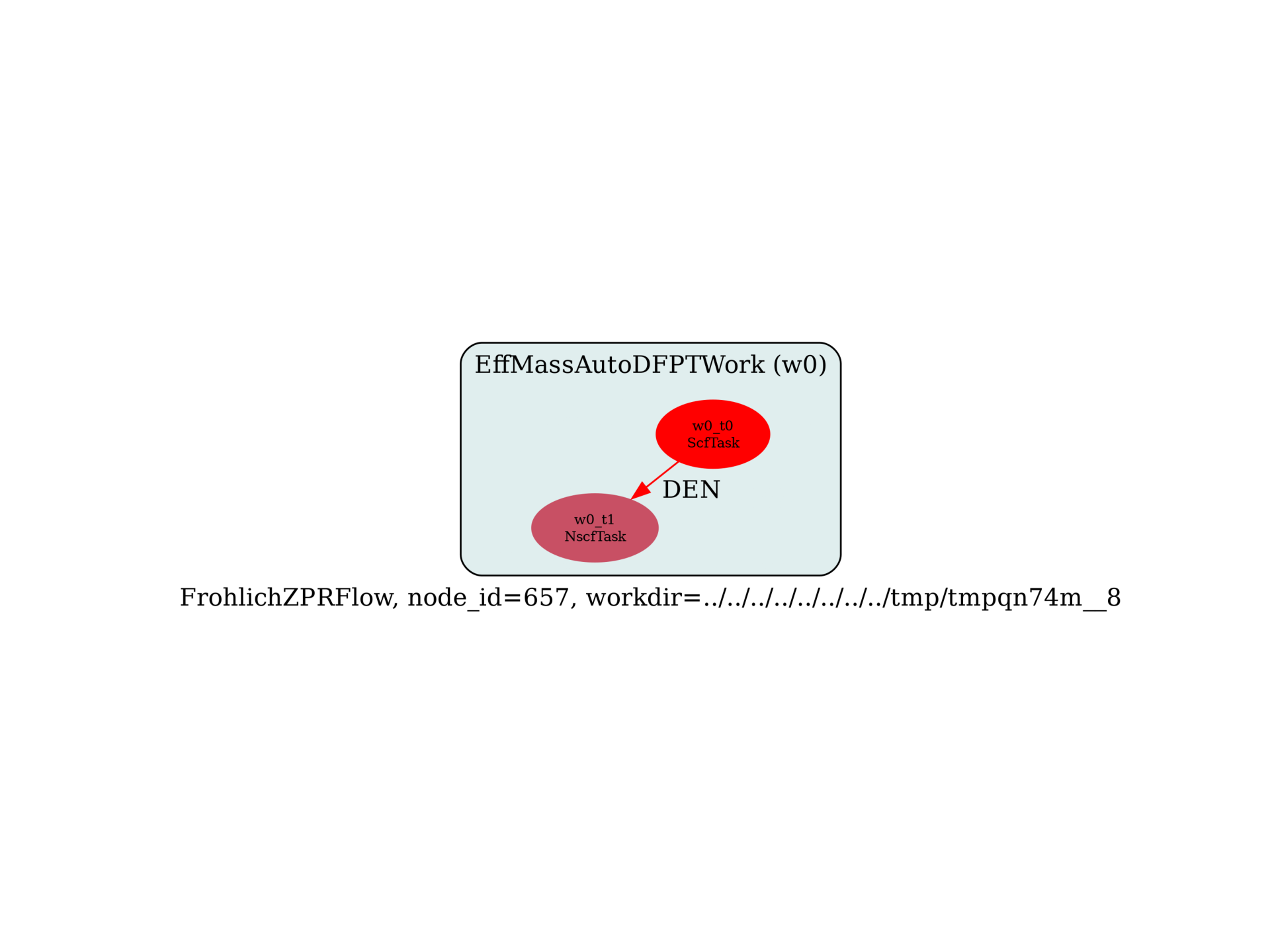Estimate the ZPR of band edges with generalized Frohlich model¶

Flow to estimate the zero-point renormalization at the band edges using the generalized Frohlich model. The flow uses DFPT to compute the effective masses at the band edges (automatically detected by performing a NSCF run with a k-path), and uses an external DDB file providing BECS, eps_inf and phonon frequencies at Gamma.

import sys
import os
import abipy.data as abidata
import abipy.abilab as abilab
import abipy.flowtk as flowtk

def build_flow(options):

# Set working directory (default is the name of the script with '.py' removed and "run_" replaced by "flow_")
if not options.workdir:
options.workdir = os.path.basename(sys.argv).replace(".py", "").replace("run_", "flow_")

# Get structure from DDB file.
ddb_path = abidata.ref_file("refs/mgo_v8t57/mgo_zpr_t57o_DS3_DDB")
with abilab.abiopen(ddb_path) as ddb:
structure = ddb.structure

# Build SCF input using structure from DDB file.
pseudos = abidata.pseudos("Ca.psp8", "O.psp8")
scf_input = abilab.AbinitInput(structure=structure, pseudos=pseudos)

# Set other input variables. These quantities are system-depedent.
# Here we use parameters similar to https://docs.abinit.org/tests/v8/Input/t57.in
scf_input.set_vars(
nband=12,
nbdbuf=2,
diemac=6,
ecut=30,                # Underconverged ecut.
#ecut=15,
nstep=100,
tolvrs=1e-16,
kptrlatt=[-2,  2,  2,   # In cartesian coordinates, this grid is simple cubic
2, -2,  2,
2,  2, -2],
)

# Build the flow to detect band edges, compute effective masses and finally obtain an estimate for the ZPR
# BECS/phonons/eps_inf are taken from ddb_node.
from abipy.flowtk.effmass_works import FrohlichZPRFlow
flow = FrohlichZPRFlow.from_scf_input(options.workdir, scf_input, ddb_node=ddb_path, ndivsm=2, tolwfr=1e-14,
manager=options.manager)
return flow

# This block generates the thumbnails in the Abipy gallery.
# You can safely REMOVE this part if you are using this script for production runs.
__name__ = None
import tempfile
options = flowtk.build_flow_main_parser().parse_args(["-w", tempfile.mkdtemp()])
build_flow(options).graphviz_imshow()

@flowtk.flow_main
def main(options):
"""
This is our main function that will be invoked by the script.
flow_main is a decorator implementing the command line interface.
Command line args are stored in `options`.
"""
return build_flow(options)

if __name__ == "__main__":
sys.exit(main())Run the script with:

run_frohlich_zpr_from_ddb.py -s

Total running time of the script: ( 0 minutes 0.457 seconds)

Gallery generated by Sphinx-Gallery